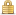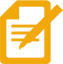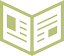## Join the FREE 5 Day Challenge Now

Get confident when running numbers and calculating cash flow on potential rental properties so you can start earning cash flow each month.We value your privacy and would never spam you

## What You'll Learn:

•Confused by all of the terms around rental property? You’ll learn all of the terms you need to know to go from rookie to veteran investor.  Learn the terms and see examples that help illustrate what each concept means.

•Knowing how to run the numbers on a deal starts with knowing what numbers you actually need to know in order to run an analysis.  We’ll show you exactly what number you need and where to find them on a real estate listing so you quickly run numbers.

•Understanding the concept of return on investment (ROI) is one of the most important elements of making smart financial decisions.  We’ll teach you what an ROI is and why you must learn to compare your cash flow to what money you invested in the property.

•This is the holy grail of the challenge.  We will show you exactly how to run the numbers on a potential deal. We’ll take the numbers you found on the listing and put them into our property analyzer so that you can see exactly what type of cash flow you can expect to return on the deal.

## Join the FREE 5 Day Challenge Now

Get confident when running numbers and calculating cash flow on potential rental properties so you can start earning cash flow each month.We value your privacy and would never spam you

## Join the FREE 5 Day Challenge Now

Get confident when running numbers and calculating cash flow on potential rental properties so you can start earning cash flow each month.We value your privacy and would never spam you